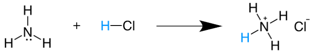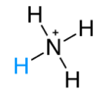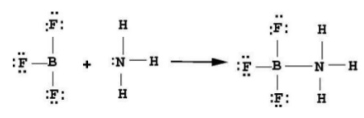# Comparative table of acid-base theories

## Table to compare acid base theories.

Acid-base theory
Definition of acid
Acid example
Definition of base
Base example
Arrhenius
A species that increases the concentration of hydrogen ions, H⁺, in an aqueous solution.
H⁺ ions react immediately with
H₂O to form oxidanium ions (H₃O⁺).
HCl(ac)H+(ac)+ Cl(ac)
Species that increases the concentration of hydroxide ions, OH, in an aqueous solution.
NaOH(ac)→ Na+(ac)OH(ac)
Brønsted-Lowry
Kind that donates a proton, H⁺.
* Water is defined as an amphoteric substance as it can act as an acid or a base. *
* Conjugated acid is the species that is formed when a Brønsted-Lowry base accepts a proton. *
NH3(g)+HCl (g) → NH4Cl (s)
* Also expressed as* Conjugated acidA species that accepts a proton, which requires a lone pair of electrons to bond to H+.
* Conjugate base is the species that forms after a Brønsted-Lowry acid gives up a proton. *
NH3(ac)+H2OR(l⇌NH4+(ac) + OH(ac)
* Conjugate base
OH(ac)
Lewis
Kind that accepts and shares
a pair of electrons given up by a base.
H++ : NH3
A species that gives up a pair of electrons and shares them with an acid.Examples of acid-base reactions according to different theories.

Reaction
Arrhenius
Brønsted-Lowry
Lewis
Commentary
HCl + NaOH → NaCl + H2O
Acid + Base → Salt + Water

HCl + NaOH → NaCl + H2O

Acid + BaseConj base + Conj acid.

HCl + NaOHNaCl + H2O

HCl + NaOH → NaCl + H2O

H2PO4 + HCl → H3PO4 + Cl
Does not apply
Acid + BaseConj acid. + Conj base

H2PO4 + HClH3PO4 + Cl

H2PO4 + HCl → H3PO4 + Cl

* Arrhenius theory does not apply because an OH ion is necessary for definition.
AlCl3 + NH3 → [Al (NH3) Cl3]
Does not apply
Does not apply